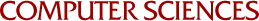## Complexity of Some Problems in Petri Nets

Neil Jones, Lawrence Landweber, Y.Edmund Lien
1976

We consider the complexity of several standard problems for various classes of Petri nets. In particular, the reachability problem, the liveness problem and the k-boundedness problems are analyzed. Some polynomial time and polynomial space complete problems for Petri nets are given. We then show that the problem of deciding whether a Petri net is persistent is reducible to reachability, partially answering a question of Keller. Reachability and boundedness are proved to be undecidable for the Time Petri net introduced by Merlin. Also presented is the concept of controllability, i.e., the capability of a set transitions to disable a given transition. We show that the controllability problem requires exponential space, even for 1-bounded nets.